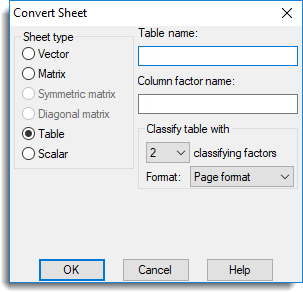1. Home
2. Convert Sheet Type

# Convert Sheet Type

Use this to convert a spreadsheet to a different type of spreadsheet.## Sheet type

Converts the spreadsheet from one type to another. The following restrictions apply to spreadsheet conversion:

• A vector spreadsheet containing text columns cannot be converted to any
other type.
• Converting a spreadsheet to type scalar will preserve row 1 and discard
all other rows.
• Spreadsheets can only be converted to diagonal or symmetric matrix type
if they are square, i.e. have equal numbers of rows and columns.
• Converting a spreadsheet to a vector type will produce columns that are
variates. These can subsequently be converted to other types using the column attributes/format menu.

## Matrix or table name

Specifies the name of a matrix or table.

## Column factor name

Specifies the name of the factor indexing the columns within a two-way table.

## Classify table with

When converting to a table this specifies how the table is to be classified.

 1 classifying factor Multiple one-way tables (one table per column) 2 classifying factors A single two-way table with a factor indexing the columns (Page format) or two factor columns and one or more table columns (Column format). 3+ classifying factors A single n-way table with a choice of page, parallel or column format.

The format of the table is controlled by the Format dropdown list.

 Page format Each table is displayed on a single page in a separate spreadsheet. The last specified classifying factor indexes the columns in the spreadsheet. Column format The tables occupy a single column but multiple tables can be put in a single spreadsheet. This is the default for a table with a single classifying factor. Tabbed format Each table is displayed on a single page in a tabbed-table spreadsheet. The separate tables appear as tabs in the spreadsheet and manipulations on one table are propagated to all other tables in the book. If there is only a single table with three or more classifying factors being saved, then the table will be displayed as a tabbed-table with the first classifying factor’s groups indexing the tabs in the book.

## Include table margins

When selected, if a table being converted to a vector or matrix sheet contains margins they will be included in the conversion, otherwise they will be removed.

## Stack table into a single variate

This option is available when converting a two or more way table, or a tabbed-table to a vector sheet. When the option is selected, all the data values in the table (after removing margins) are placed into a single variate column along with factors indexing the tables values. The variate and factors have their names automatically generated based on the original table information. If the sheet is part of a tabbed book, then all the data from the sheets are placed into the vector sheet.

## Close original spreadsheet on conversion

This option only appears when the Stack table into a single variate option has been selected. When selected the original table spreadsheet used to create the new vector spreadsheet will be closed.

## Tabbed table has separate tables on each page

When selected, a table converted using the Tabbed format will create tabs in the book with separate tables on each page. Alternatively, the tab will be a classifying factor of a table that contains all the data in the book.

In the command language, a variate or factor can be converted to a text with the PRINT directive:
PRINT [CHANNEL=TXT; IPRINT=*; SQUASH=yes] VAR

A variate or text can be converted to a factor with the GROUPS directive:
GROUPS VAR; FACTOR=FACT
GROUPS [MODIFY=yes] VAR “if the same structure is to be changed”

A text can be converted to a variate with a READ directive:

In the command language, one-way table can be converted to variates using the TPROJECT calculate function:
CALCULATE VAR = TPROJECT(Table)

In the command language, a two-way table can be converted to a matrix using the TMATRIX calculate function:
CALCULATE MAT = TMATRIX(Table;RowFactor;ColFactor)

The EQUATE directive can also be used to move data from one structure to another:
VARIATE  V[1…10]
EQUATE OLDSTRUCTURE=Matrix; NEWSTRUCTURE=V
MATRIX [10;4] Matrix2
EQUATE OLDSTRUCTURE=!p(A,B,C,D); NEWSTRUCTURE=Matrix2

Updated on March 20, 2019